# 100 100 200 200 300 300 400 400

• Slides: 72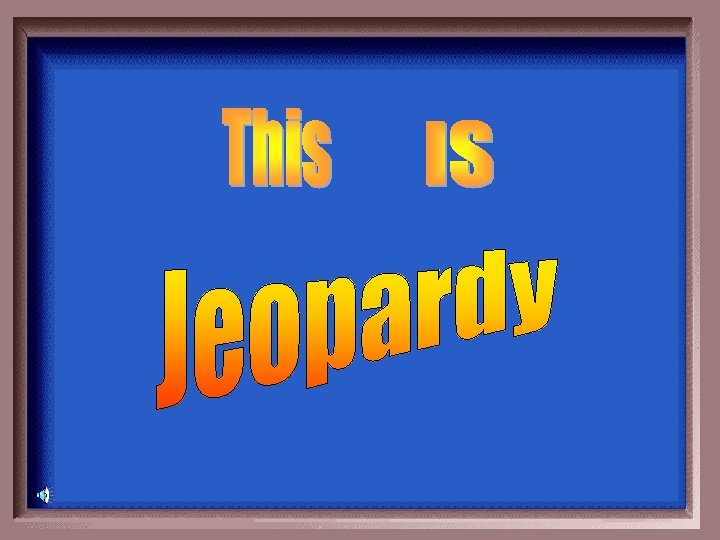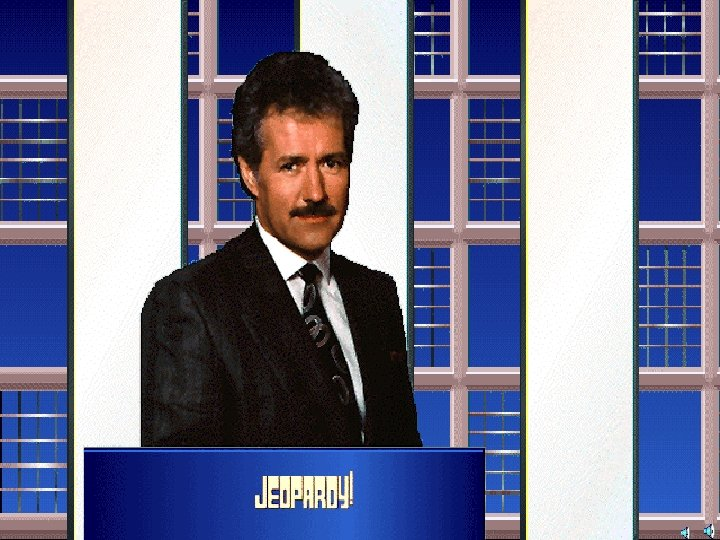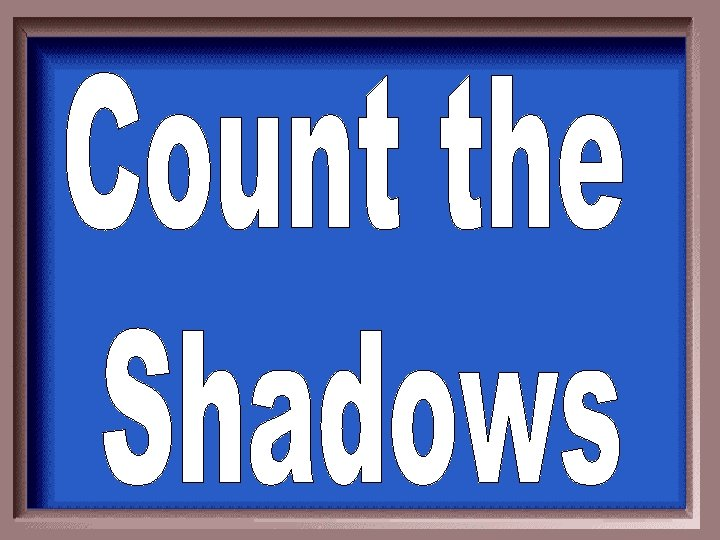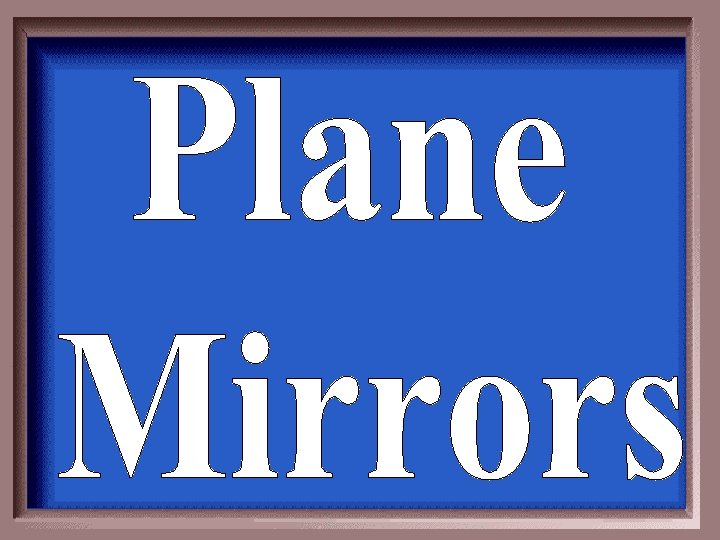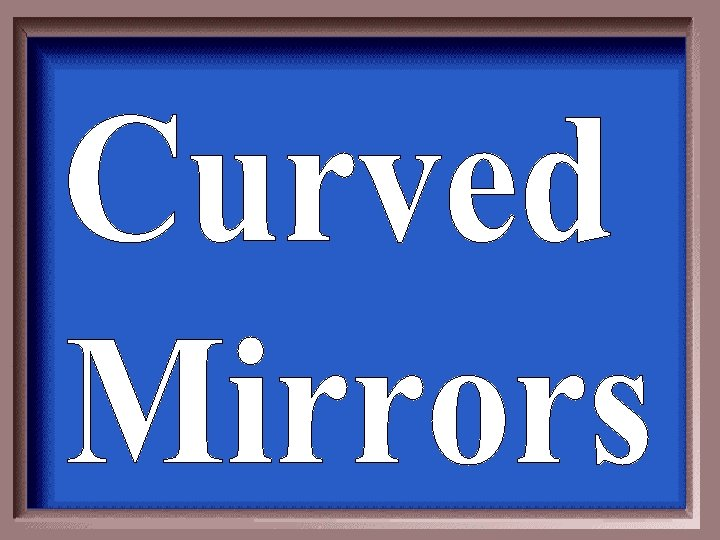\$100 \$100 \$200 \$200 \$300 \$300 \$400 \$400 \$500 \$500Draw a ray.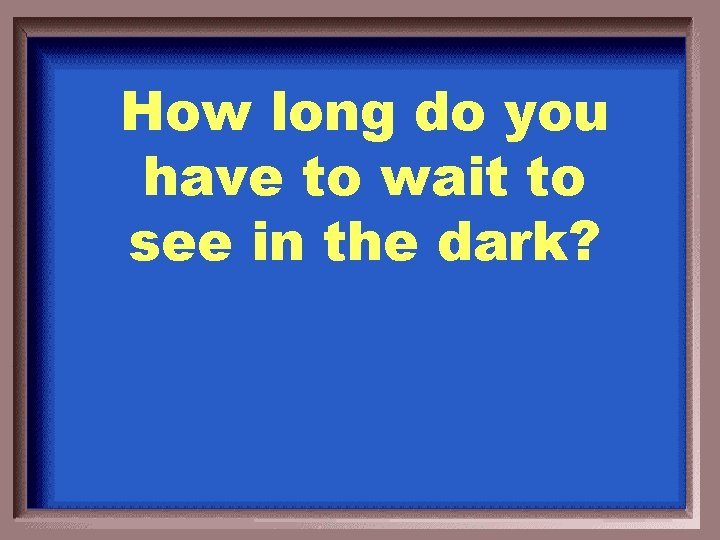How long do you have to wait to see in the dark?Never will be able to see, nothing emittedDescribe the conditions necessary to see lightHas to reflect off an object and into your eyes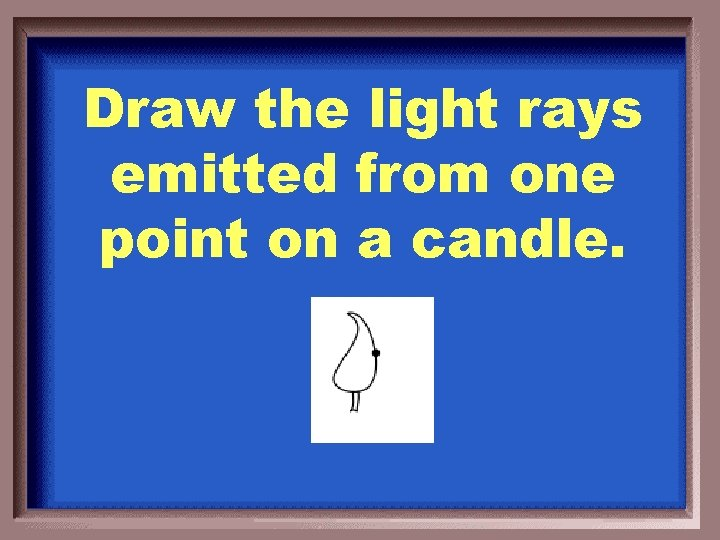Draw the light rays emitted from one point on a candle.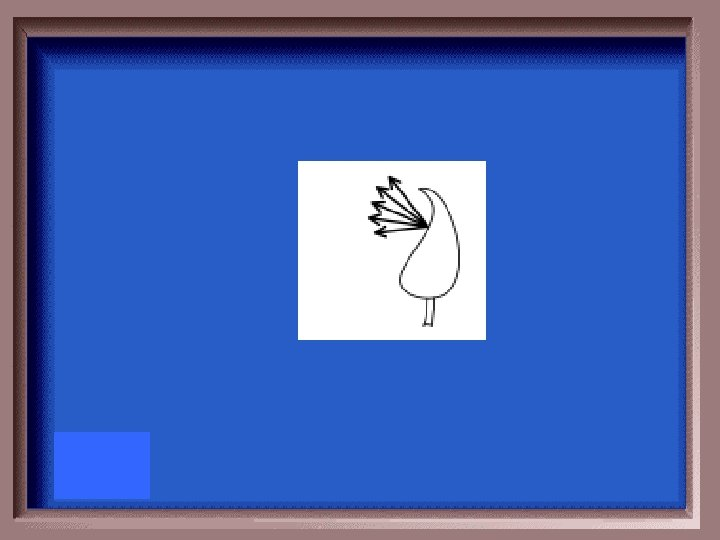How does a pinhole work? Draw the object and image.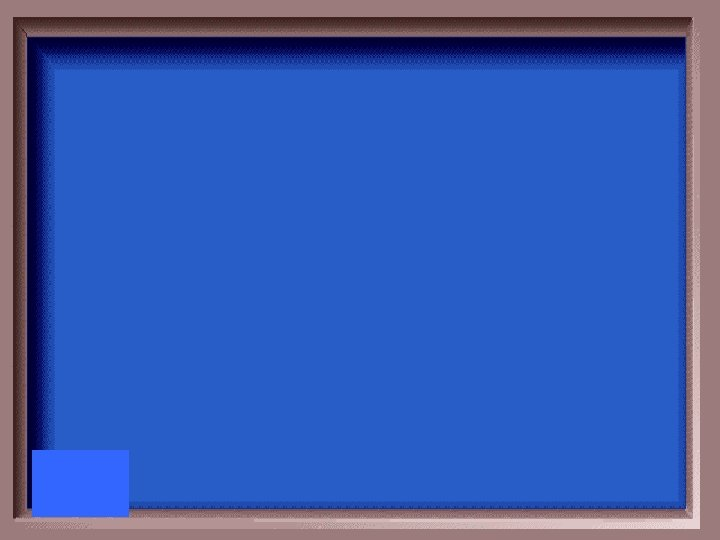What causes the formation of shadows?When light shines on an object, some of the rays may be stopped while others pass on in a straight-line path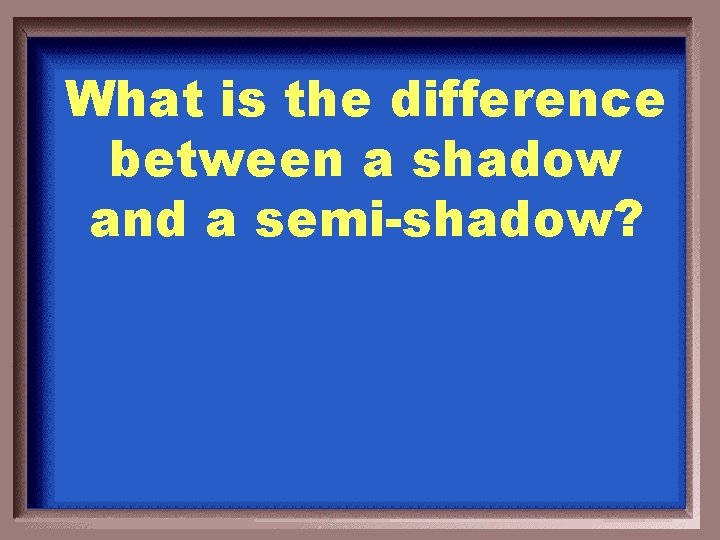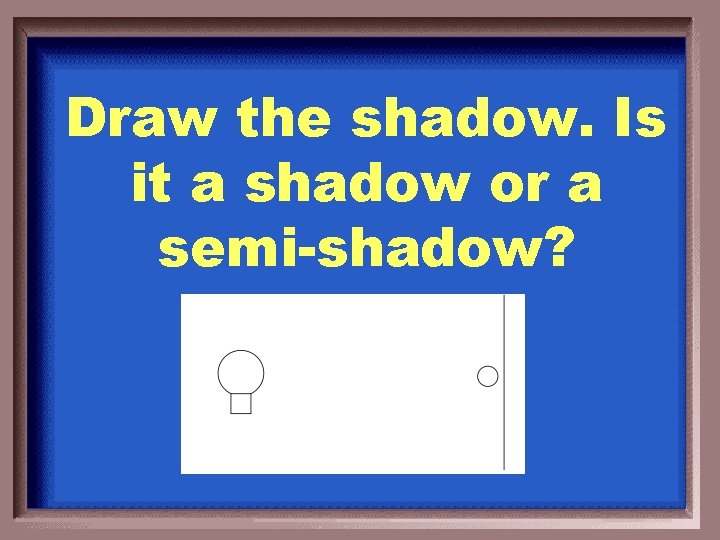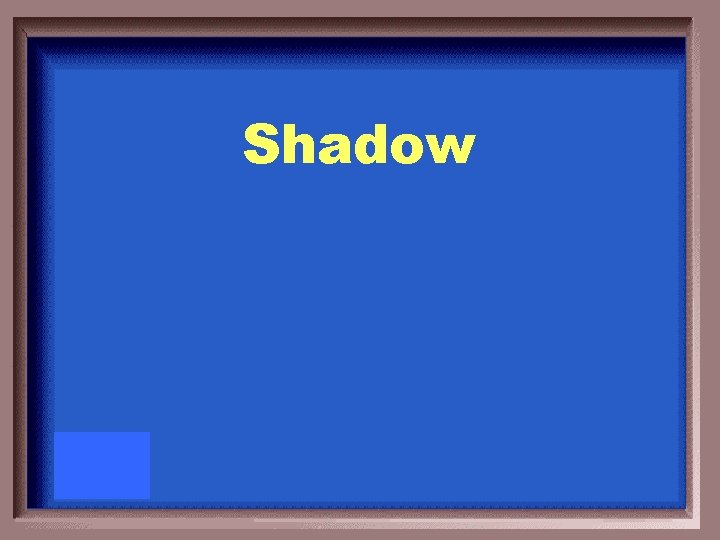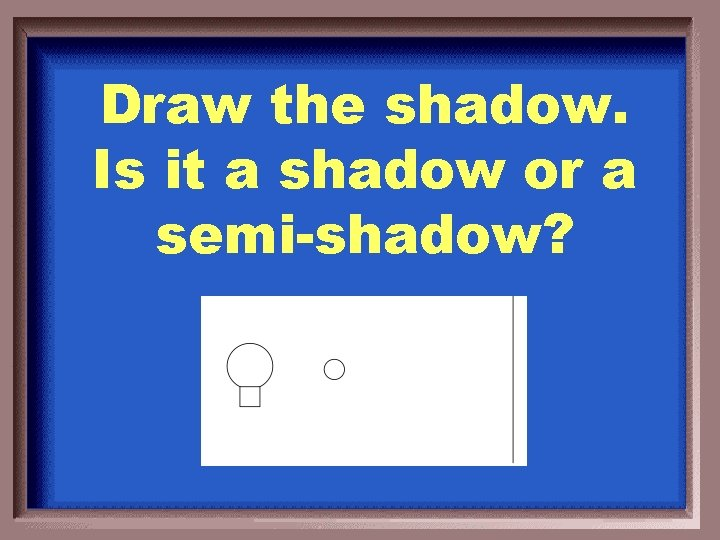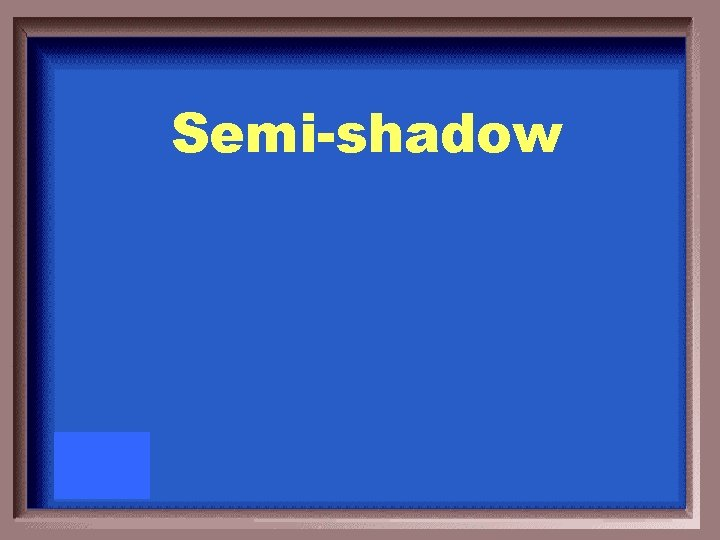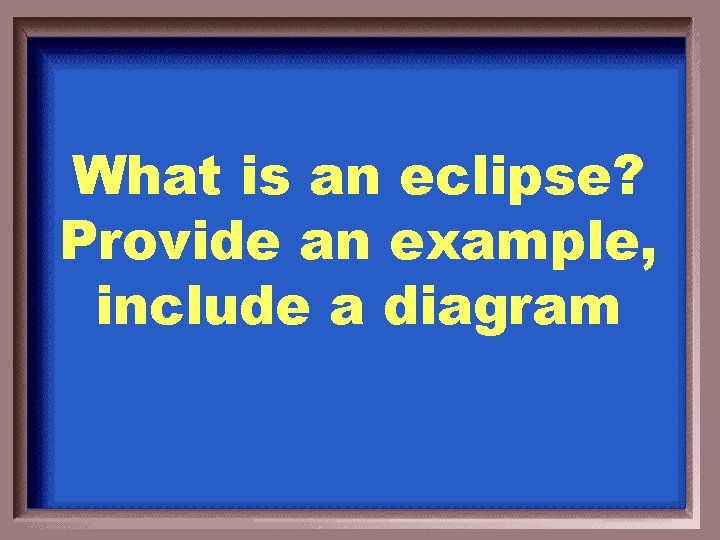What is an eclipse? Provide an example, include a diagramSolar eclipse: moon passes between earth and sun Lunar eclipse: earth passes between sun and moonWhat is the law of reflection?Angle of incidence = angle of reflection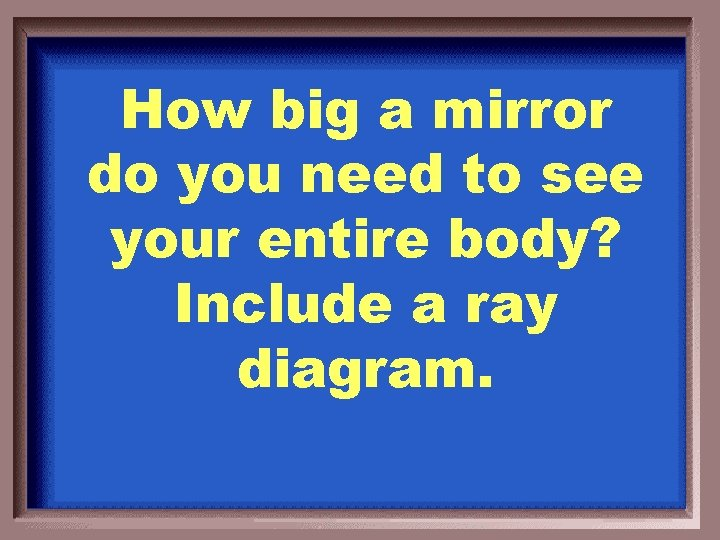How big a mirror do you need to see your entire body? Include a ray diagram.A mirror half your height (diagram on board)What is the normal? Why is it so important?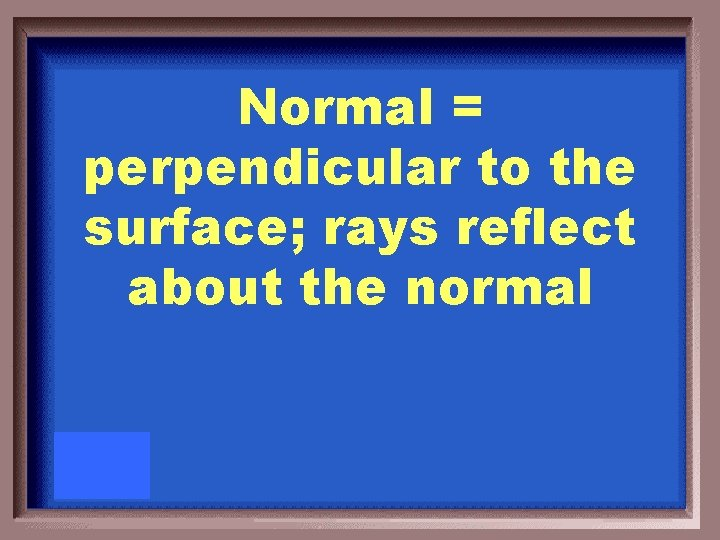Normal = perpendicular to the surface; rays reflect about the normalA 50 cm dog stands 3 m from a plane mirror and looks at its image. Draw a ray diagram for the dog. Describe the image.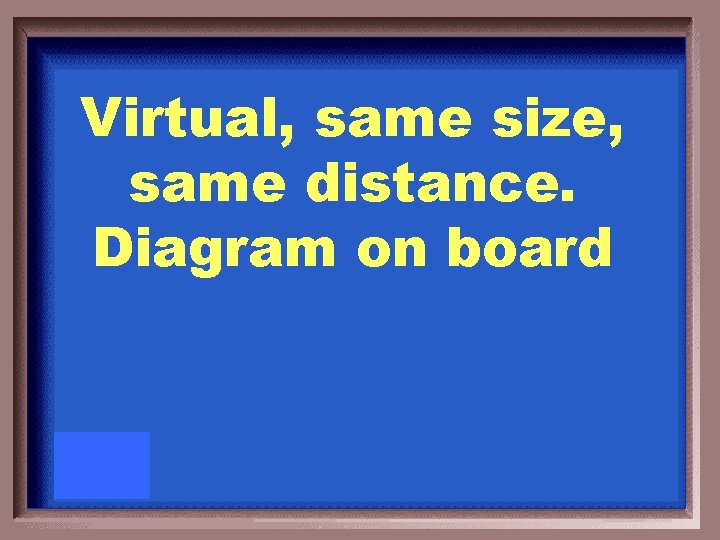Virtual, same size, same distance. Diagram on boardDescribe the process of triangulation. Where is it used in your everyday life? Why is it necessary for our study of optics?Triangulation = using multiple observers to pinpoint a source (ex: image location for a plane mirror, gps, satellite tv, etc)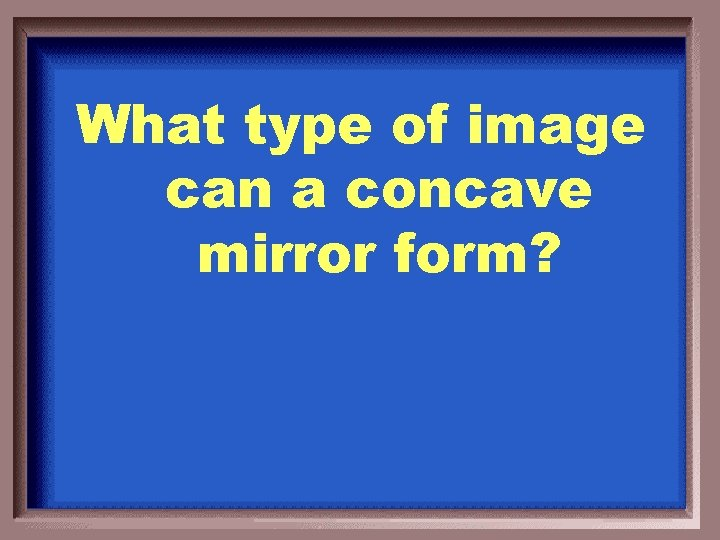What type of image can a concave mirror form?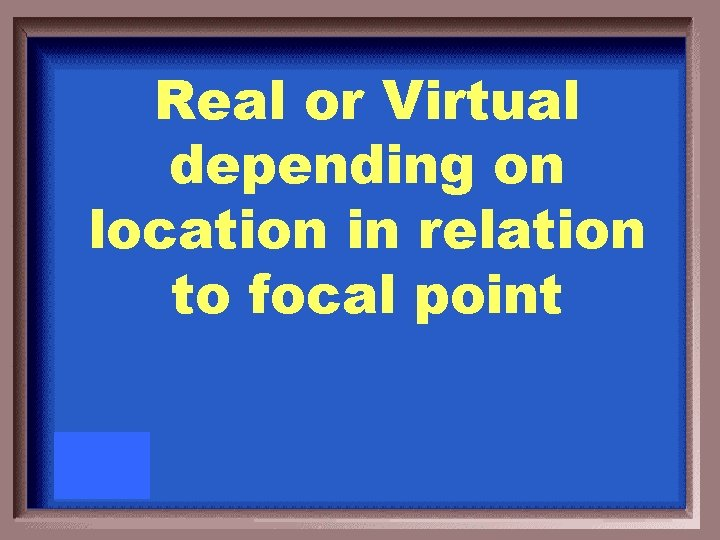Real or Virtual depending on location in relation to focal pointWhere is the focal point located for a convex mirror? What type of image is always formed?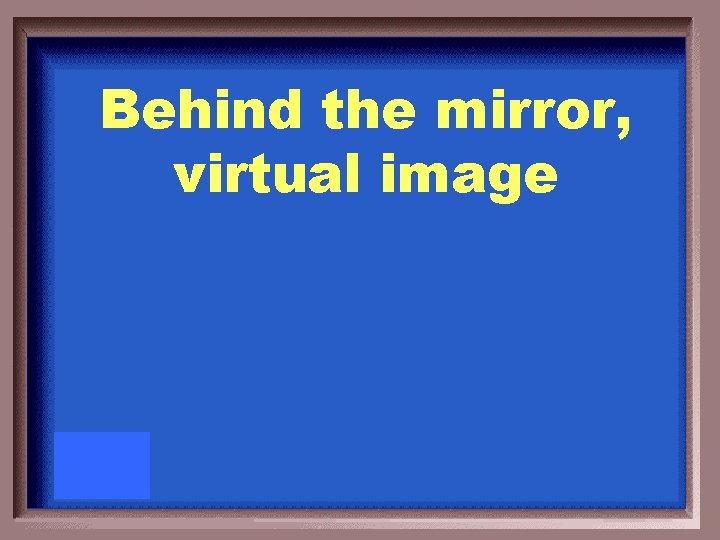Behind the mirror, virtual imageWhat type of mirror is this? Why is there a warning?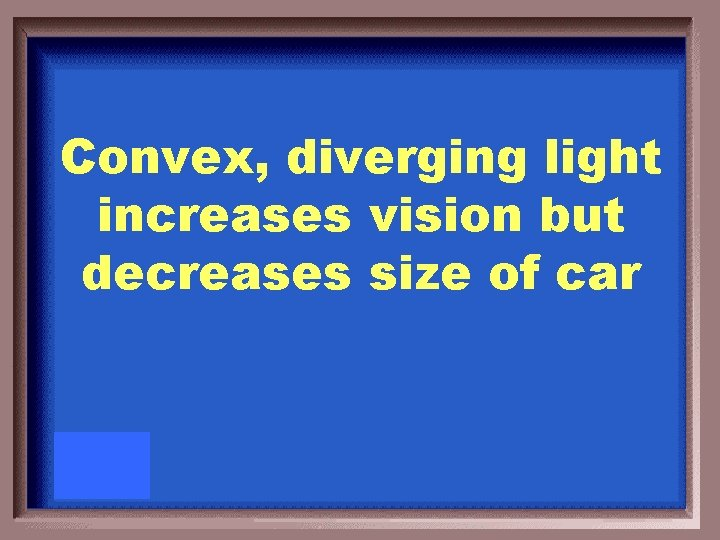Convex, diverging light increases vision but decreases size of carLocate the position, orientation, and type of image formed by an upright object held in front of a concave mirror of focal length +10 cm. The object distance is 54 cm and is 10 cm tall. Include a ray diagram.di = 12. 27 cm M = - 0. 23 hi= -2. 3 cm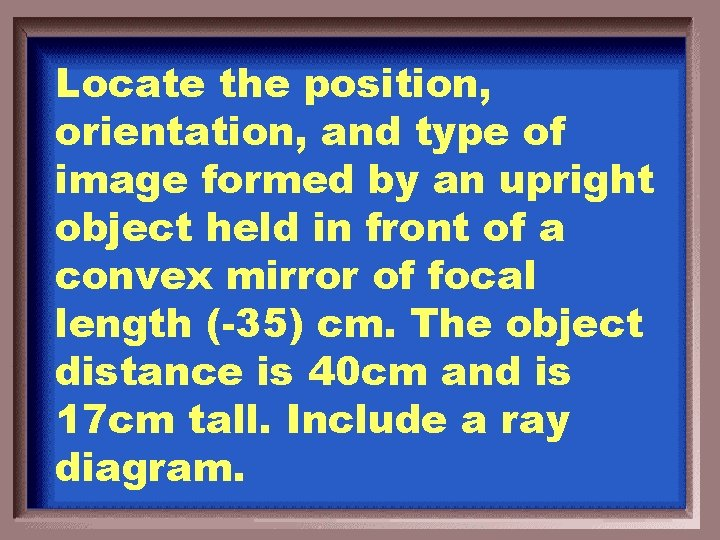Locate the position, orientation, and type of image formed by an upright object held in front of a convex mirror of focal length (-35) cm. The object distance is 40 cm and is 17 cm tall. Include a ray diagram.di = -18. 66 cm M = 0. 46 hi= 7. 93 cmDescribe how light bends as it changes mediums.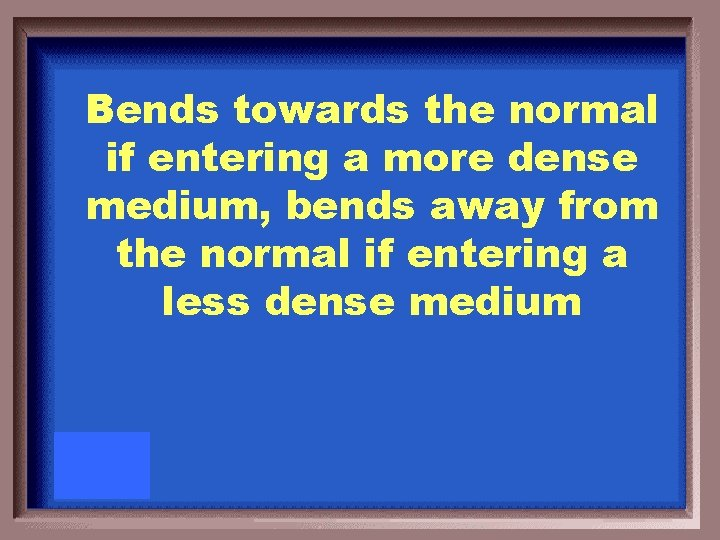Bends towards the normal if entering a more dense medium, bends away from the normal if entering a less dense mediumHeather is snorkeling in Oahu’s Hanauma Bay when she looks up through the water at a palm tree on the shore. If the index of refraction of the water is 1. 33 and Heather sees the palm tree at an angle of 45 degrees, at what angle is the palm tree really located with respect to the normal? Include a diagram.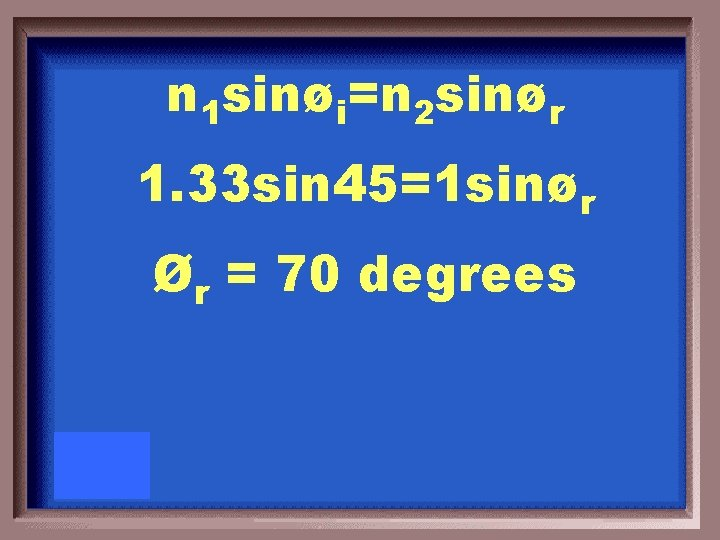n 1 sinøi=n 2 sinør 1. 33 sin 45=1 sinør Ør = 70 degrees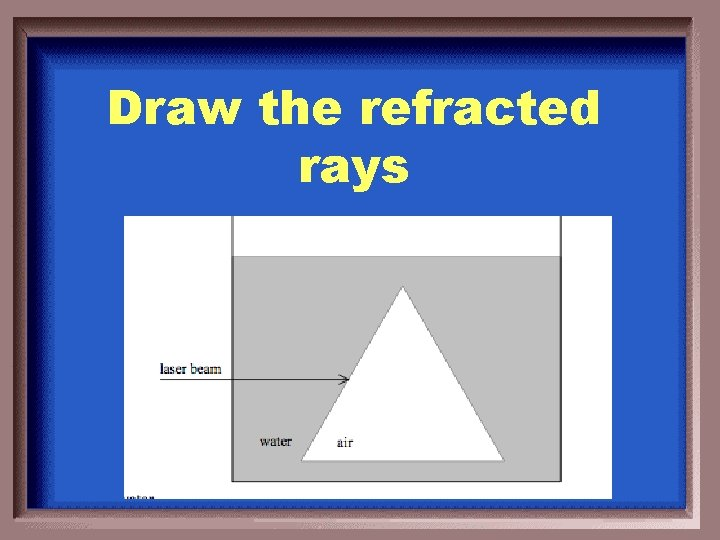Draw the refracted rays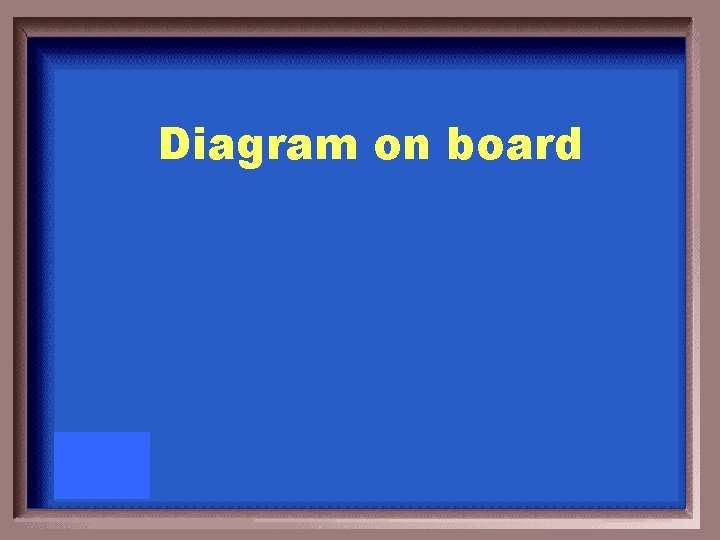Diagram on boardHow do rainbows form? Draw a ray diagram!Different wavelengths of colored light refract differently, becomes trapped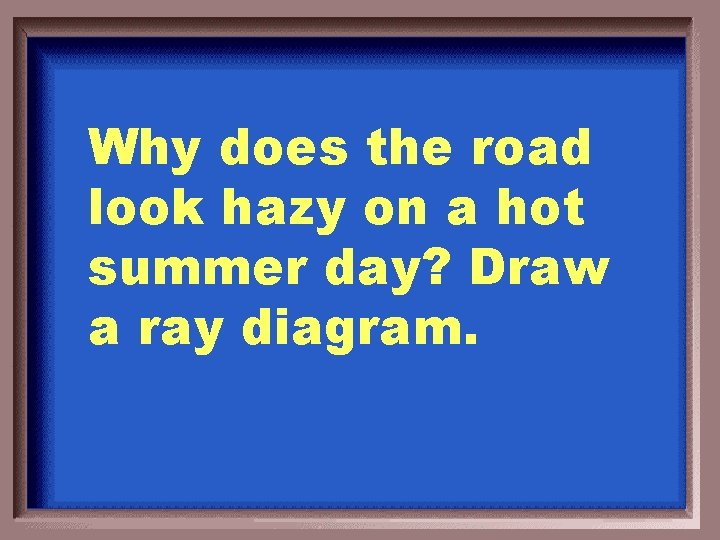Why does the road look hazy on a hot summer day? Draw a ray diagram.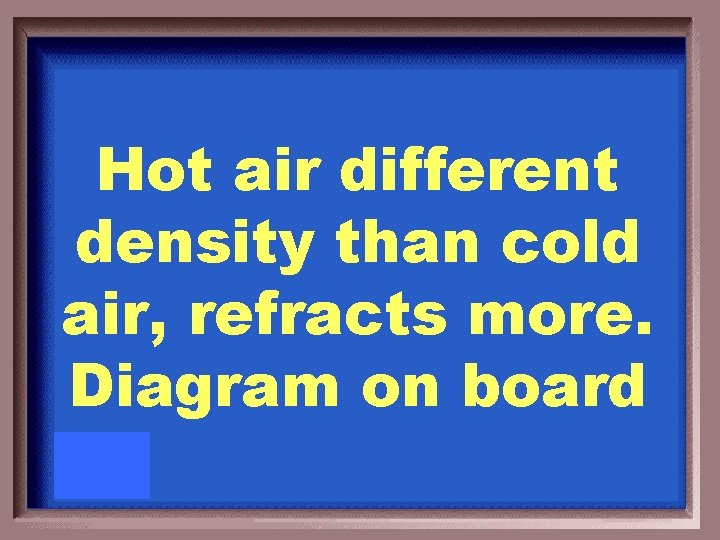Hot air different density than cold air, refracts more. Diagram on boardWhat is the difference between concave and convex lenses?Concave = diverging Convex = converging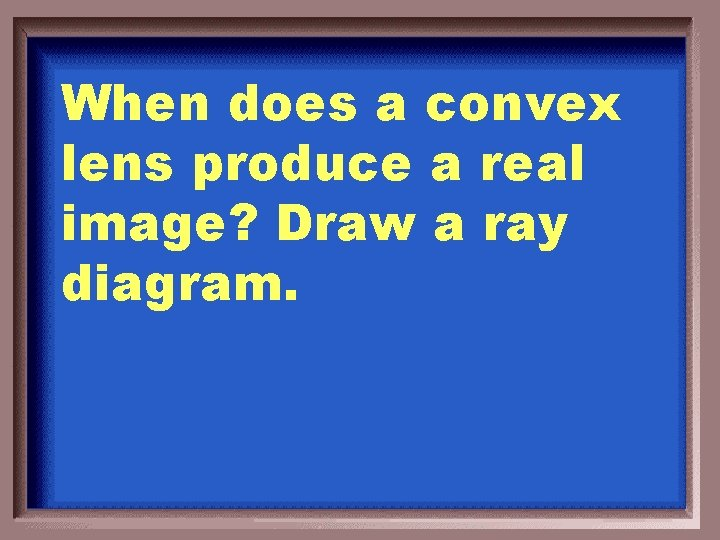When does a convex lens produce a real image? Draw a ray diagram.When the object is located behind the focal point.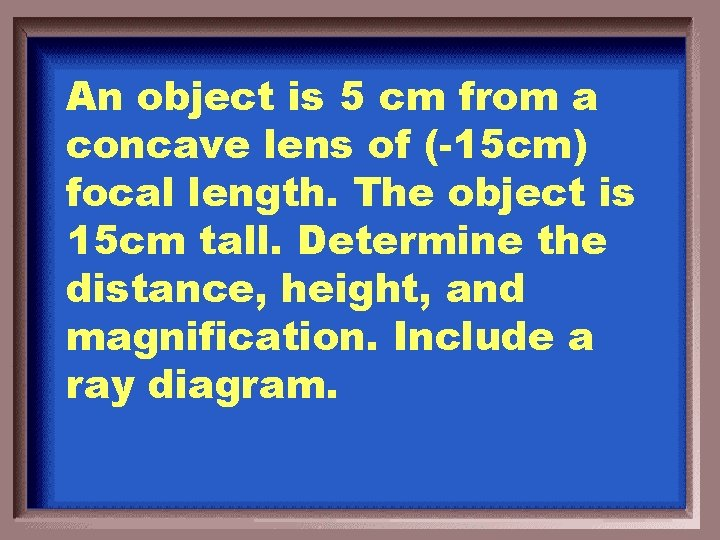An object is 5 cm from a concave lens of (-15 cm) focal length. The object is 15 cm tall. Determine the distance, height, and magnification. Include a ray diagram.di = -3. 75 cm M = 0. 75 hi= 11. 25 cm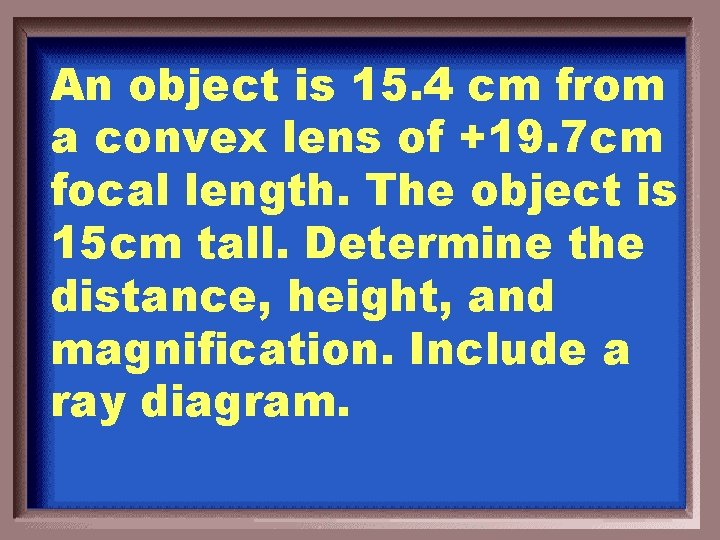An object is 15. 4 cm from a convex lens of +19. 7 cm focal length. The object is 15 cm tall. Determine the distance, height, and magnification. Include a ray diagram.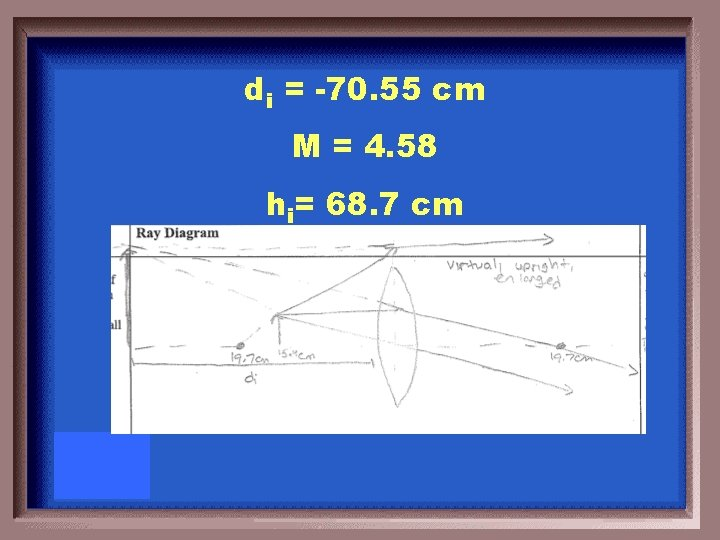di = -70. 55 cm M = 4. 58 hi= 68. 7 cmDescribe how the human eye works. What does it mean to be nearsighted? To be farsighted? Draw a ray diagram for each.Nearsighted: cannot see far Farsighted: cannot see near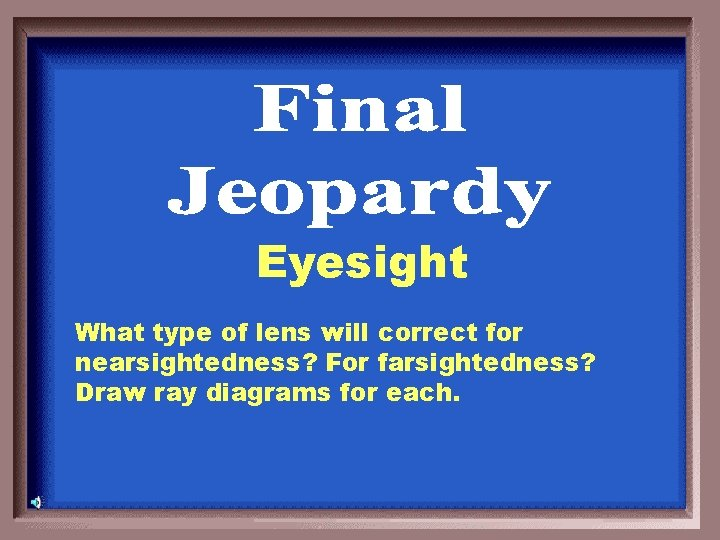Eyesight What type of lens will correct for nearsightedness? For farsightedness? Draw ray diagrams for each.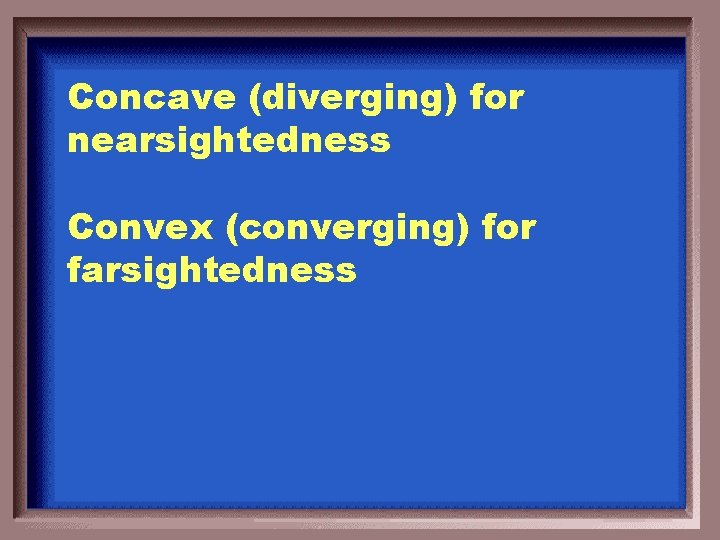Concave (diverging) for nearsightedness Convex (converging) for farsightedness